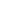#Decimal Numbers MTH-1006 is a Course

## Decimal Numbers MTH-1006

Self-paced

### Full course description

Course Description

If you work with measuring instruments or engineering drawings, then you’ve probably encountered numbers that contain decimals. Fortunately, working with decimals isn’t rocket science, so you can quickly master the basics of adding, subtracting, multiplying, and dividing decimals.

By the end of this course, you will be able to

• Identify place values of decimal numbers

• Understand the power of tens

• Determine when to use leading and trailing zeros

• Add, subtract, multiply, and divide decimals

• Round decimal numbers

Estimated completion time (hours): 1.0